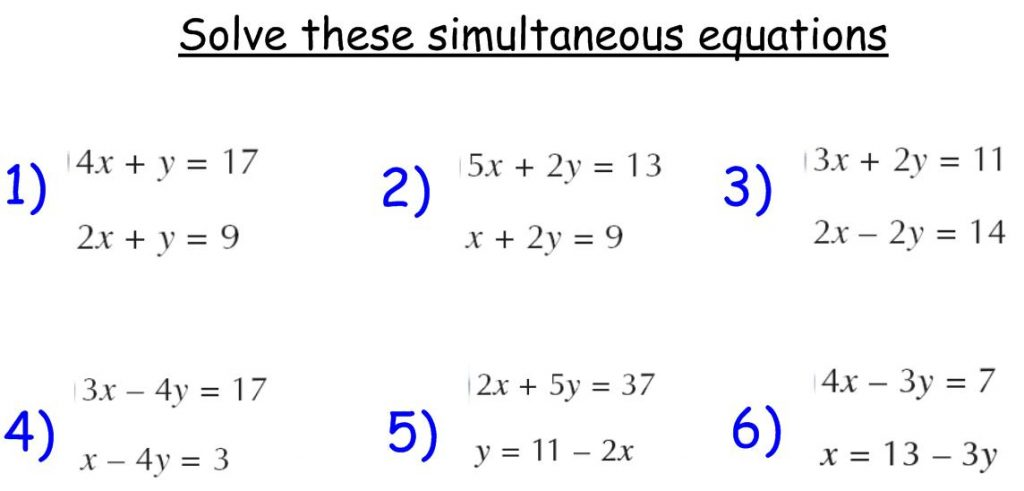# SOLVING SIMULTANEOUS EQUATIONS USING SUBSTITUTION METHOD 2 (VIDEO)Watch the video here on how to solve simultaneous equations using substitution method:

After watching the video, solve the following equations using substitution method:

4x + 3y  =  10  …………….(i)

3x + 5y  = 13  ………………(ii)